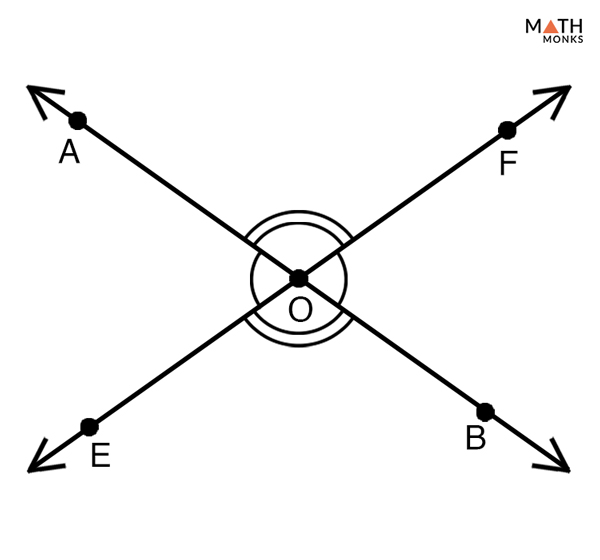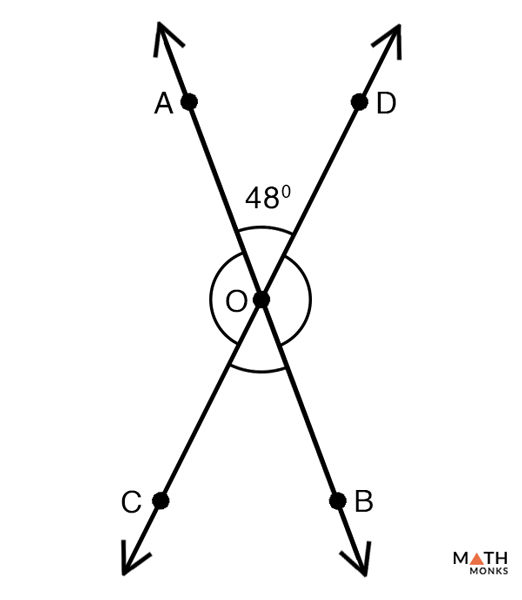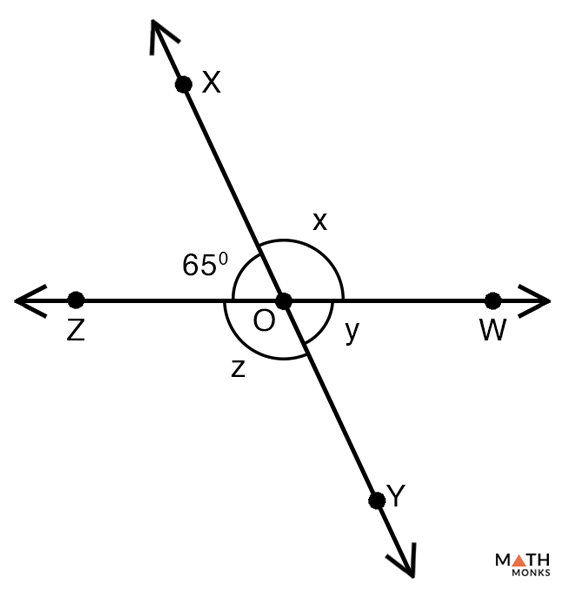# Vertical Angles

## What are Vertical Angles

Vertical angles are pairs of opposite angles formed when two lines intersect each other at a point. They are thus also known as vertically opposite angles. Any two intersecting lines form two pairs of vertical angles. In geometry, the word ‘vertical’ means ‘related to a vertex’ or corner.

The above figure shows two pairs of vertically opposite angles that are equal.

## Vertical Angles TheoremProve Vertical Angles Theorem

To prove:

∠AOE = ∠BOF, ∠AOF = ∠BOE

Proof:

Given that, AB and EF are the two line segments that intersect at point ‘O’
Now, ∠AOF and ∠BOF form a linear pair and thus they are supplementary
∴∠AOF + ∠BOF = 180°……. (1)
Similarly, ∠AOF = ∠AOE form another linear pair and are also supplementary
∴ ∠AOF + ∠AOE = 180°……. (2)
From (1) and (2) we get,
∠AOF + ∠BOF = ∠AOF + ∠AOE
Subtracting ∠AOF from both sides we get,
∠BOF = ∠AOE…… (3)
Similarly,
∠BOF and ∠ BOE form a linear pair and are supplementary
∠BOF + ∠ BOE = 180°…….. (4)
From (1) and (4) we get,
∠AOF + ∠BOF = ∠BOF + ∠BOE
Subtracting ∠BOF from both sides we get,
∠AOF = ∠BOE…….. (5)
From (3) and (5) we can say that vertical angles are always congruent or equal to each other.
Hence Proved

## How to Solve Vertical Angles

Let us find some vertical angles in the given examples to understand the concept better.Name the pair of vertical angles in the given figure and find the values of the unknown angles.

Solution:

By definition, in the above figure, ∠AOD, ∠BOC and ∠DOB, ∠COA are the two pair of vertical angles
Thus,
∠AOD = ∠BOC
∠DOB = ∠COA
Given, ∠AOD = 48°
Hence,
∠BOC = 48°
Now,
∠AOD + ∠DOB = 180° (linear pair of supplementary angles)
∠BOC +∠COA = 180° (linear pair of supplementary angles)
Now,
∠AOD + ∠DOB = 180°, here ∠AOD = 48°
48° + ∠DOB = 180°
∠DOB = 180° – 48°
∠DOB = 132°
Since, ∠DOB = ∠COA (Vertical angles)
∠COA = 132°Consider the figure given alongside to identify the pair of vertical angles and find their values.

Solution:

In the given figure,
∠WOX = ∠ZOY (vertical angles)
∠XOZ = ∠WOY (vertical angles)
Now, given ∠XOZ = 65°
Thus, ∠WOY = y = 65°
Again, ∠XOZ + ∠WOX = 180° (linear pair), here ∠XOZ = 65°, ∠WOX = x
65° + x = 180°
x = 180°- 65°
x = 115°
Similarly,
∠ZOY + ∠WOY = 180° (linear pair), here ∠WOY = 65°, ∠ZOY = z
z + 65° = 180°
z = 180° – 65°
z = 115°
Alternative method
In the given figure,
∠WOX = ∠ZOY (vertical angles)
∠XOZ = ∠WOY (vertical angles)
Now, given ∠XOZ = 65°
Thus, ∠WOY = y = 65°
Again, ∠XOZ + ∠WOX = 180° (linear pair), here ∠XOZ = 65°, ∠WOX = x
65° + x = 180°
x = 180°- 65°
x = 115°
Since,
∠WOX = ∠ZOY (Vertical angles)
∠ZOY = z = 115°

## Examples in Real Life

• Opened scissor
• Alphabet X
• Two wooden sticks meet in a kite

## FAQs

Q1. Can Vertical Angles be Adjacent?

Ans. By definition, vertical angels cannot be adjacent or next to each other. This is because the second pair of vertical angles interrupts in their way of becoming adjacent.  Adjacent angles take one angle from one pair of vertical angles and another angle from another pair.

Q2. Are Vertical Angles Supplementary?

Ans. As we know, supplementary angles add up to 180°, vertical angles can only be supplementary when the two lines intersect perpendicularly. Thus vertical angles are not always supplementary.

Q3. Are Vertical Angles Complementary?

Ans. Since complementary angles add up to 90°, vertical angles can only be complementary when each of them are acute and measures 45°. Thus acute vertical angles can be complementary when they measure 45°.